Bohr Model of an Atom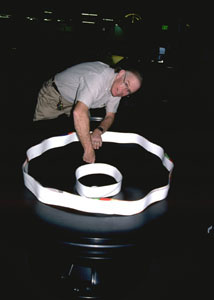Paul Doherty places rings containing 1 complete wave and 2 complete waves inside an energy well modelling the energy well of an atom.

Introduction

Use waves drawn on paper strips to model the wave function of an electron then use this to model the energy levels of an atom.
This exploration uses a large gravity well usually found in science museums.

Material

• Cash register tape or other paper tape, you'll need 3.5 meters (or 14 ft.)
• optional, black plastic flexible hose, white spray paint
• felt tip marker pen
• Sticky tape
• A gravity well The gravity well is the same shape as the electrical energy well for an electron near a proton.
So we can use the gravity well to model an atom.
• Optional a "Vortx coin bank" sold by Educational Innovations.(yes it is spelled Vortx)
• Optional. Mount the paper with the -1/x curve drawn on it on a wall. Hold up the circles and find a place where the circles just fit inside the -1/x curve as shown in the opening image above.

Assembly

For the museum size gravity well use the following length waves.

Cut one paper strip 25 cm long ( a foot).

Draw a sine wave on this paper, then roll this paper into a circle. Call this circle number 1.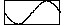Cut a 1 meter ( 4 ft.) strip and draw two wavelengths on it. Roll the strip into a circle and tape it together. Call this number 2.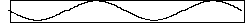Cut a 2.2 meter (9 ft.) strip and draw three wavelengths on it. Roll it into a circle and call it number 2.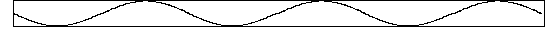Optional assembly for the Vortex Coin Bank

For the coin bank use the following wavelengths instead: 6 inches for the single wave, 2 ft for the double wave, the triple wave will not fit but it would be 54 inches long.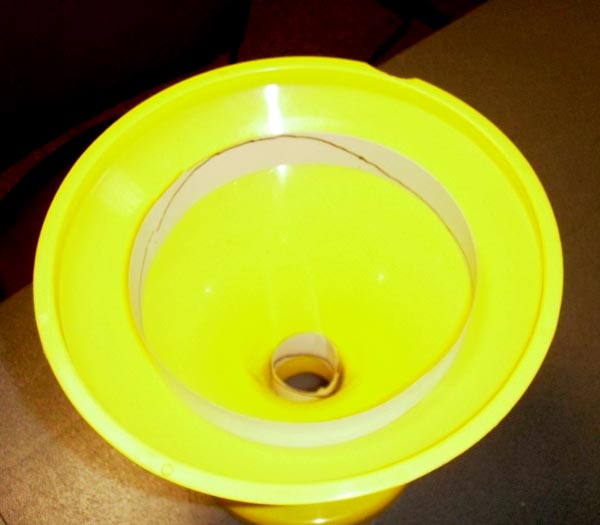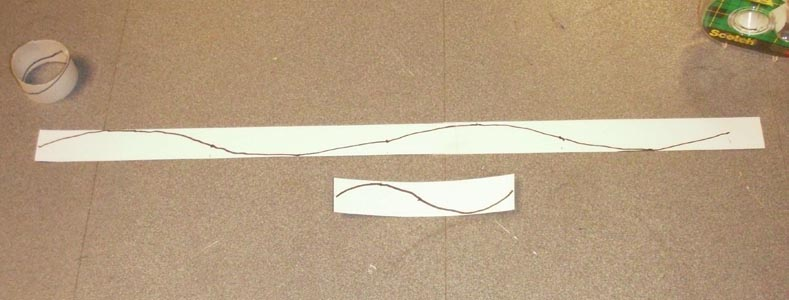The electron waves wrapped into a circle

Optional more rugged assembly

Cut pieces of hose to the same length as the paper strips and tape them together into a circle (you can also insert a short cylinder inside one end of the hose, leaving some sticking out, then slide the other end over the protruding bit. Paint half of the smallest circle white, paint alternating fourths of the middle length circle white, and paint alternating sixths of the longest piece white. The hose keeps its circular shape better than the paper.

To Do and Notice.

Take the smallest circle and drop it into the gravity well. It drops down until one wavelength exactly fits around the circumference of the gravity well.

Do the same for the other two circles.

Notice the pattern formed by the circles.

Notice the pattern of lengths, 1,4,9, it is the pattern of squares of integers.

What's Going On?

Did you ever wonder why the negatively charged electron doesn't just fall toward the positively charged proton in a hydrogen atom?

The answer to this question using classical physics from the 19'th century is that the electron does fall toward the nucleus. But it misses! This is the essence of an orbit. Just as the moon falls toward the earth because of gravity, and yet is moving to the side so that it never strikes the earth, so the electron orbits the proton.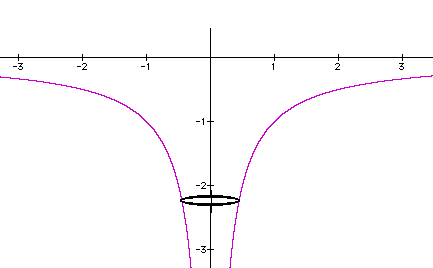The potential energy curve for an electron orbiting a proton.
Plus a circle representing an orbit.

However, there was another question, when an electron orbits in a circle it emits electromagnetic radiation.
This radiation removes energy from the orbiting electron causing it to spiral into the proton in a millisecond or so.

The Bohr model used the quantum mechanical concept that an electron travels as a probability wave.
The wavelength of the electron wave depends on the velocity (and so the kinetic energy) of the electron, higher energy electrons have shorter wavelengths.

If the electron orbits an atom as a wave, then something special happens when one wave exactly fits around an orbit.
This standing wave does not lose energy by radiation.
The same thing happens when two, three or any integer number of waves fit around the atom.

These standing waves define the energy levels and diameters of the orbits of electrons around protons.

The standing wave condition allowed the Bohr model to explain the energy levels in the hydrogen atom.

Math Root

There are several related mathematical bits needed here.

The potential energy, V, of the electron of charge, -e, around a nucleus with charge, Ze, as a function of distance, r, is:

V = - kZe2/r

The smaller the radius the larger the depth of the electrical potential energy. Cut the radius in half and the depth of the potential energy curve doubles.

where k = 1/4pe0 the coulomb constant, 9 x 109 N-m2/C2

For hydrogen where Z = 1, we can group all the constant terms together in parentheses and find V = -(kZe2)/r.

The kinetic energy of an electron is KE = (1/2) mv2

Where m is the mass of the electron.

But in a circular orbit the velocity must have a value so that the centripetal force is provided by electrical attraction.
The magnitude of the electric force is the classic inverse square law of Coulomb. F = kZe2/r2
The magnitude of the centripetal force needed to hold a mass in a circular orbit is, F = mv2/r

set these equal and solve for v2 then use v2 to find kinetic energy.

kZe2/r2 = mv2/r

So the kinetic energy as a function of radius is: KE = (1/2) kZe2/r

The kinetic energy is opposite in sign to the potential energy and has half the magnitude.
This is true for all circular orbits in inverse square force fields.

The total energy as a function of radius is:

E = V + KE = -(1/2) kZe2/r

To change radius a particle must gain or lose energy,
i.e. if r changes then the total energy E must change.

The interesting thing is that there are certain orbital radii which are stable in atoms. To understand these we must learn about the wave properties of electrons.

An electron travels as a wave, a wave of probability density, that is, a wave in the probability of finding and electron.

The relationship between the kinetic energy of an electron, KE, and its wavelength, L

L = h/(2mKE)0.5

Where h is Planck's constant, 6.6 x 10-34 Js.

So the wavelength is inversely proportional to the square root of the energy.

If an integer number of waves fit in a circle about the nucleus then

nL = 2pr

substituting for L

nh/(2mKE)0.5 = 2p r

KE = n2h2/(2m4p2 r2)

Now to find the radius at which there are n wavelengths in the circumference of the orbit, set the two equations for KE equal and find.

n2h2/(2m4p2 r2) = (1/2) kZe2/r

solve for r

r = (h2/4p2ke2)n2/Z

I've gathered all the constant stuff in the parentheses.

The neat stuff outside the parentheses shows that the radius increases as the square of the number of wavelengths, n2, and decreases inversely with the nuclear charge Z.

The energy levels are inversely proportional to r so the potential energy, En, of level n is

En = -(4p2mk2e4/2h2)Z2/n2

So for hydrogen if we call the energy of the first energy level, with n=1, E1 = -1

Then the next energy level E2 = -1/4

and the next E3 = -1/9.

This is close to what is actually observed and was a triumph for the Bohr model.

Going Further

Sine waves are very useful curves. Mr. Fourier discovered that they can be added together to create periodic functions of any shape. However, in three dimensions the basic shapes of nature needed to assemble all rotationally symmetric shapes were more complicated. The spherical Legendre Polynomials. Schrodinger used these basic shapes in three dimensions to more accurately model the wave functions of electrons around atoms. These are the orbitals used to explain energy levels in modern quantum mechanics.